# Mean State

Period Mean (original grids) [Watt m-2]
Model Period Mean (intersection) [Watt m-2]
Model Period Mean (complement) [Watt m-2]
Benchmark Period Mean (intersection) [Watt m-2]
Benchmark Period Mean (complement) [Watt m-2]
Bias [Watt m-2]
RMSE [Watt m-2]
Phase Shift [months]
Bias Score 
RMSE Score 
Seasonal Cycle Score 
Spatial Distribution Score 
Interannual Variability Score 
Overall Score 
Benchmark [-] 38.3
CRUNCEPv7 [-] 36.3 36.0 0.00 39.6 15.5 -3.01 10.6 1.70 0.38 0.37 0.72 0.95 0.48 0.55
GSWP3v1 [-] 33.9 33.6 0.00 39.6 15.5 -5.32 11.4 1.57 0.31 0.37 0.75 0.98 0.53 0.55
WATCH [-] 26.4 26.1 0.00 39.8 15.2 -12.9 17.5 2.43 0.13 0.33 0.59 0.92 0.53 0.47
Period Mean (original grids) [Watt m-2]
Model Period Mean (intersection) [Watt m-2]
Model Period Mean (complement) [Watt m-2]
Benchmark Period Mean (intersection) [Watt m-2]
Benchmark Period Mean (complement) [Watt m-2]
Bias [Watt m-2]
RMSE [Watt m-2]
Phase Shift [months]
Bias Score 
RMSE Score 
Seasonal Cycle Score 
Spatial Distribution Score 
Interannual Variability Score 
Overall Score 
Benchmark [-] 52.3
CRUNCEPv7 [-] 47.2 47.6 0.00 52.8 14.1 -5.21 13.3 1.35 0.44 0.39 0.83 0.99 0.53 0.60
GSWP3v1 [-] 45.3 45.8 0.00 52.8 14.1 -7.07 13.8 1.27 0.40 0.38 0.85 0.99 0.53 0.59
WATCH [-] 37.4 37.9 0.00 53.3 14.1 -15.4 20.5 1.45 0.20 0.34 0.82 0.98 0.49 0.53
Period Mean (original grids) [Watt m-2]
Model Period Mean (intersection) [Watt m-2]
Model Period Mean (complement) [Watt m-2]
Benchmark Period Mean (intersection) [Watt m-2]
Benchmark Period Mean (complement) [Watt m-2]
Bias [Watt m-2]
RMSE [Watt m-2]
Phase Shift [months]
Bias Score 
RMSE Score 
Seasonal Cycle Score 
Spatial Distribution Score 
Interannual Variability Score 
Overall Score 
Benchmark [-] 39.3
CRUNCEPv7 [-] 36.9 36.9 0.00 40.4 16.6 -3.30 11.9 1.76 0.41 0.41 0.73 0.91 0.56 0.57
GSWP3v1 [-] 36.6 36.6 0.00 40.4 16.6 -3.70 12.2 1.81 0.40 0.38 0.72 0.91 0.58 0.56
WATCH [-] 33.4 33.4 0.00 40.6 16.5 -7.14 14.5 2.01 0.28 0.37 0.69 0.96 0.57 0.54
Period Mean (original grids) [Watt m-2]
Model Period Mean (intersection) [Watt m-2]
Model Period Mean (complement) [Watt m-2]
Benchmark Period Mean (intersection) [Watt m-2]
Benchmark Period Mean (complement) [Watt m-2]
Bias [Watt m-2]
RMSE [Watt m-2]
Phase Shift [months]
Bias Score 
RMSE Score 
Seasonal Cycle Score 
Spatial Distribution Score 
Interannual Variability Score 
Overall Score 
Benchmark [-] 31.6
CRUNCEPv7 [-] 36.8 36.6 0.00 31.5 32.6 6.48 20.3 0.340 0.77 0.58 0.98 0.88 0.71 0.75
GSWP3v1 [-] 34.3 34.2 0.00 31.5 32.6 3.60 18.0 0.327 0.80 0.59 0.98 0.97 0.76 0.78
WATCH [-] 33.8 33.7 0.00 32.0 33.8 2.53 17.9 0.275 0.82 0.60 0.98 0.98 0.78 0.79
Period Mean (original grids) [Watt m-2]
Model Period Mean (intersection) [Watt m-2]
Model Period Mean (complement) [Watt m-2]
Benchmark Period Mean (intersection) [Watt m-2]
Benchmark Period Mean (complement) [Watt m-2]
Bias [Watt m-2]
RMSE [Watt m-2]
Phase Shift [months]
Bias Score 
RMSE Score 
Seasonal Cycle Score 
Spatial Distribution Score 
Interannual Variability Score 
Overall Score 
Benchmark [-] 23.5
CRUNCEPv7 [-] 29.7 29.6 0.00 25.9 15.5 4.21 11.0 2.04 0.43 0.38 0.68 0.20 0.43 0.42
GSWP3v1 [-] 27.8 27.7 0.00 25.9 15.5 2.49 11.1 1.43 0.36 0.36 0.79 0.23 0.55 0.44
WATCH [-] 22.2 22.1 0.00 25.5 15.5 -2.07 12.0 1.97 0.24 0.34 0.70 0.69 0.59 0.48
Period Mean (original grids) [Watt m-2]
Model Period Mean (intersection) [Watt m-2]
Model Period Mean (complement) [Watt m-2]
Benchmark Period Mean (intersection) [Watt m-2]
Benchmark Period Mean (complement) [Watt m-2]
Bias [Watt m-2]
RMSE [Watt m-2]
Phase Shift [months]
Bias Score 
RMSE Score 
Seasonal Cycle Score 
Spatial Distribution Score 
Interannual Variability Score 
Overall Score 
Benchmark [-] 21.4
CRUNCEPv7 [-] 25.5 25.4 0.00 21.5 17.6 3.83 10.8 0.499 0.73 0.52 0.94 0.87 0.70 0.71
GSWP3v1 [-] 25.4 25.3 0.00 21.5 17.6 3.82 12.6 0.569 0.73 0.47 0.93 0.82 0.74 0.69
WATCH [-] 21.5 21.4 0.00 21.3 18.0 0.134 11.1 0.586 0.77 0.49 0.93 0.84 0.71 0.70
Period Mean (original grids) [Watt m-2]
Model Period Mean (intersection) [Watt m-2]
Model Period Mean (complement) [Watt m-2]
Benchmark Period Mean (intersection) [Watt m-2]
Benchmark Period Mean (complement) [Watt m-2]
Bias [Watt m-2]
RMSE [Watt m-2]
Phase Shift [months]
Bias Score 
RMSE Score 
Seasonal Cycle Score 
Spatial Distribution Score 
Interannual Variability Score 
Overall Score 
Benchmark [-] 30.0
CRUNCEPv7 [-] 39.2 39.1 0.00 30.5 14.9 7.88 13.8 1.08 0.55 0.45 0.86 0.97 0.66 0.65
GSWP3v1 [-] 35.3 35.3 0.00 30.5 14.9 4.16 11.4 0.960 0.67 0.47 0.87 0.97 0.67 0.69
WATCH [-] 35.2 35.2 0.00 29.8 15.1 4.69 13.7 1.18 0.63 0.42 0.84 0.89 0.68 0.65
Period Mean (original grids) [Watt m-2]
Model Period Mean (intersection) [Watt m-2]
Model Period Mean (complement) [Watt m-2]
Benchmark Period Mean (intersection) [Watt m-2]
Benchmark Period Mean (complement) [Watt m-2]
Bias [Watt m-2]
RMSE [Watt m-2]
Phase Shift [months]
Bias Score 
RMSE Score 
Seasonal Cycle Score 
Spatial Distribution Score 
Interannual Variability Score 
Overall Score 
Benchmark [-] 29.5
CRUNCEPv7 [-] 37.6 37.6 0.00 30.6 14.2 6.13 12.4 0.935 0.55 0.41 0.89 0.98 0.48 0.62
GSWP3v1 [-] 33.5 33.4 0.00 30.6 14.2 2.29 11.6 0.992 0.59 0.40 0.88 0.98 0.51 0.63
WATCH [-] 30.6 30.5 0.00 29.2 14.1 0.872 12.3 1.13 0.49 0.37 0.86 0.94 0.53 0.59
Period Mean (original grids) [Watt m-2]
Model Period Mean (intersection) [Watt m-2]
Model Period Mean (complement) [Watt m-2]
Benchmark Period Mean (intersection) [Watt m-2]
Benchmark Period Mean (complement) [Watt m-2]
Bias [Watt m-2]
RMSE [Watt m-2]
Phase Shift [months]
Bias Score 
RMSE Score 
Seasonal Cycle Score 
Spatial Distribution Score 
Interannual Variability Score 
Overall Score 
Benchmark [-] 33.0
CRUNCEPv7 [-] 35.1 34.9 0.00 32.8 37.1 2.86 19.3 0.271 0.81 0.60 0.98 0.98 0.65 0.77
GSWP3v1 [-] 32.3 32.2 0.00 32.8 37.1 -0.0780 17.2 0.288 0.82 0.61 0.98 0.85 0.73 0.77
WATCH [-] 30.4 30.2 0.00 33.2 38.1 -2.44 16.0 0.234 0.86 0.63 0.98 0.92 0.68 0.78
Period Mean (original grids) [Watt m-2]
Model Period Mean (intersection) [Watt m-2]
Model Period Mean (complement) [Watt m-2]
Benchmark Period Mean (intersection) [Watt m-2]
Benchmark Period Mean (complement) [Watt m-2]
Bias [Watt m-2]
RMSE [Watt m-2]
Phase Shift [months]
Bias Score 
RMSE Score 
Seasonal Cycle Score 
Spatial Distribution Score 
Interannual Variability Score 
Overall Score 
Benchmark [-] 35.1
CRUNCEPv7 [-] 40.8 40.8 0.00 36.7 14.1 3.07 10.8 0.654 0.58 0.53 0.95 0.90 0.64 0.69
GSWP3v1 [-] 39.7 39.8 0.00 36.7 14.1 2.08 10.6 0.510 0.58 0.53 0.96 0.89 0.65 0.69
WATCH [-] 40.4 40.4 0.00 36.2 14.0 3.09 10.7 0.625 0.57 0.52 0.94 0.96 0.76 0.71
Period Mean (original grids) [Watt m-2]
Model Period Mean (intersection) [Watt m-2]
Model Period Mean (complement) [Watt m-2]
Benchmark Period Mean (intersection) [Watt m-2]
Benchmark Period Mean (complement) [Watt m-2]
Bias [Watt m-2]
RMSE [Watt m-2]
Phase Shift [months]
Bias Score 
RMSE Score 
Seasonal Cycle Score 
Spatial Distribution Score 
Interannual Variability Score 
Overall Score 
Benchmark [-] 19.8
CRUNCEPv7 [-] 26.8 26.9 0.00 20.7 10.0 5.61 11.4 1.02 0.62 0.52 0.89 0.97 0.68 0.70
GSWP3v1 [-] 22.5 22.5 0.00 20.7 10.0 1.77 10.1 1.13 0.71 0.53 0.87 0.87 0.68 0.70
WATCH [-] 19.3 19.3 0.00 21.0 10.1 -1.72 10.6 1.05 0.66 0.53 0.87 0.85 0.69 0.69
Period Mean (original grids) [Watt m-2]
Model Period Mean (intersection) [Watt m-2]
Model Period Mean (complement) [Watt m-2]
Benchmark Period Mean (intersection) [Watt m-2]
Benchmark Period Mean (complement) [Watt m-2]
Bias [Watt m-2]
RMSE [Watt m-2]
Phase Shift [months]
Bias Score 
RMSE Score 
Seasonal Cycle Score 
Spatial Distribution Score 
Interannual Variability Score 
Overall Score 
Benchmark [-] 23.6
CRUNCEPv7 [-] 47.6 47.6 0.00 43.1 14.1 3.75 16.6 0.898 0.59 0.48 0.89 0.88 0.56 0.65
GSWP3v1 [-] 45.0 45.0 0.00 43.1 14.1 1.14 14.4 0.858 0.62 0.49 0.89 0.88 0.60 0.66
WATCH [-] 42.6 42.6 0.00 42.8 14.3 -0.881 15.8 0.985 0.57 0.47 0.87 0.90 0.61 0.65
Period Mean (original grids) [Watt m-2]
Model Period Mean (intersection) [Watt m-2]
Model Period Mean (complement) [Watt m-2]
Benchmark Period Mean (intersection) [Watt m-2]
Benchmark Period Mean (complement) [Watt m-2]
Bias [Watt m-2]
RMSE [Watt m-2]
Phase Shift [months]
Bias Score 
RMSE Score 
Seasonal Cycle Score 
Spatial Distribution Score 
Interannual Variability Score 
Overall Score 
Benchmark [-] 64.3
CRUNCEPv7 [-] 81.5 81.3 0.00 66.2 16.5 13.1 21.0 0.697 0.53 0.55 0.93 0.98 0.53 0.68
GSWP3v1 [-] 78.4 78.2 0.00 66.2 16.5 10.2 18.6 0.552 0.56 0.57 0.95 0.99 0.54 0.70
WATCH [-] 70.5 70.3 0.00 65.0 16.0 3.63 17.0 0.592 0.60 0.56 0.94 0.98 0.53 0.70
Period Mean (original grids) [Watt m-2]
Model Period Mean (intersection) [Watt m-2]
Model Period Mean (complement) [Watt m-2]
Benchmark Period Mean (intersection) [Watt m-2]
Benchmark Period Mean (complement) [Watt m-2]
Bias [Watt m-2]
RMSE [Watt m-2]
Phase Shift [months]
Bias Score 
RMSE Score 
Seasonal Cycle Score 
Spatial Distribution Score 
Interannual Variability Score 
Overall Score 
Benchmark [-] 34.3
CRUNCEPv7 [-] 42.0 41.9 0.00 34.2 35.0 9.42 24.2 0.362 0.71 0.56 0.97 0.95 0.68 0.74
GSWP3v1 [-] 37.5 37.5 0.00 34.2 35.0 4.47 18.3 0.207 0.79 0.61 0.98 0.98 0.77 0.79
WATCH [-] 38.8 38.7 0.00 34.6 35.8 5.42 21.9 0.205 0.77 0.56 0.98 0.98 0.78 0.77
Period Mean (original grids) [Watt m-2]
Model Period Mean (intersection) [Watt m-2]
Model Period Mean (complement) [Watt m-2]
Benchmark Period Mean (intersection) [Watt m-2]
Benchmark Period Mean (complement) [Watt m-2]
Bias [Watt m-2]
RMSE [Watt m-2]
Phase Shift [months]
Bias Score 
RMSE Score 
Seasonal Cycle Score 
Spatial Distribution Score 
Interannual Variability Score 
Overall Score 
Benchmark [-] 24.8
CRUNCEPv7 [-] 34.1 34.1 0.00 27.0 14.3 6.78 11.3 0.907 0.48 0.45 0.90 0.79 0.54 0.60
GSWP3v1 [-] 33.8 33.8 0.00 27.0 14.3 6.58 11.1 0.872 0.52 0.44 0.91 0.85 0.59 0.62
WATCH [-] 32.2 32.2 0.00 27.6 14.7 4.02 11.4 1.07 0.48 0.41 0.88 0.97 0.61 0.63
Period Mean (original grids) [Watt m-2]
Model Period Mean (intersection) [Watt m-2]
Model Period Mean (complement) [Watt m-2]
Benchmark Period Mean (intersection) [Watt m-2]
Benchmark Period Mean (complement) [Watt m-2]
Bias [Watt m-2]
RMSE [Watt m-2]
Phase Shift [months]
Bias Score 
RMSE Score 
Seasonal Cycle Score 
Spatial Distribution Score 
Interannual Variability Score 
Overall Score 
Benchmark [-] 20.7
CRUNCEPv7 [-] 30.7 30.6 0.00 21.1 12.1 9.05 12.2 1.02 0.42 0.45 0.86 0.93 0.65 0.63
GSWP3v1 [-] 27.4 27.3 0.00 21.1 12.1 6.00 9.62 0.994 0.56 0.48 0.87 0.99 0.65 0.67
WATCH [-] 30.0 29.9 0.00 22.1 12.4 7.36 12.8 1.32 0.51 0.40 0.80 0.82 0.68 0.60
Period Mean (original grids) [Watt m-2]
Model Period Mean (intersection) [Watt m-2]
Model Period Mean (complement) [Watt m-2]
Benchmark Period Mean (intersection) [Watt m-2]
Benchmark Period Mean (complement) [Watt m-2]
Bias [Watt m-2]
RMSE [Watt m-2]
Phase Shift [months]
Bias Score 
RMSE Score 
Seasonal Cycle Score 
Spatial Distribution Score 
Interannual Variability Score 
Overall Score 
Benchmark [-] 20.3
CRUNCEPv7 [-] 26.2 26.3 0.00 20.6 10.6 5.71 11.9 0.662 0.61 0.51 0.92 0.83 0.64 0.67
GSWP3v1 [-] 23.7 23.7 0.00 20.6 10.6 3.05 10.4 0.622 0.68 0.53 0.92 0.90 0.67 0.70
WATCH [-] 23.4 23.5 0.00 20.9 11.2 2.65 11.6 0.683 0.66 0.49 0.91 0.86 0.67 0.68
Period Mean (original grids) [Watt m-2]
Model Period Mean (intersection) [Watt m-2]
Model Period Mean (complement) [Watt m-2]
Benchmark Period Mean (intersection) [Watt m-2]
Benchmark Period Mean (complement) [Watt m-2]
Bias [Watt m-2]
RMSE [Watt m-2]
Phase Shift [months]
Bias Score 
RMSE Score 
Seasonal Cycle Score 
Spatial Distribution Score 
Interannual Variability Score 
Overall Score 
Benchmark [-] 30.8
CRUNCEPv7 [-] 36.0 36.0 0.00 31.1 10.8 4.52 10.7 0.919 0.63 0.50 0.87 0.99 0.72 0.70
GSWP3v1 [-] 34.2 34.2 0.00 31.1 10.8 2.76 9.44 0.757 0.70 0.51 0.90 0.99 0.73 0.73
WATCH [-] 38.0 38.1 0.00 31.2 11.1 6.44 12.7 1.39 0.57 0.44 0.78 0.89 0.72 0.64
Period Mean (original grids) [Watt m-2]
Model Period Mean (intersection) [Watt m-2]
Model Period Mean (complement) [Watt m-2]
Benchmark Period Mean (intersection) [Watt m-2]
Benchmark Period Mean (complement) [Watt m-2]
Bias [Watt m-2]
RMSE [Watt m-2]
Phase Shift [months]
Bias Score 
RMSE Score 
Seasonal Cycle Score 
Spatial Distribution Score 
Interannual Variability Score 
Overall Score 
Benchmark [-] 26.8
CRUNCEPv7 [-] 28.8 28.8 0.00 27.1 15.2 1.84 8.42 1.63 0.69 0.37 0.77 0.71 0.42 0.55
GSWP3v1 [-] 27.1 27.1 0.00 27.1 15.2 0.130 8.33 2.25 0.74 0.37 0.64 0.81 0.45 0.56
WATCH [-] 23.7 23.7 0.00 28.6 15.4 -4.77 10.5 2.06 0.53 0.35 0.68 0.84 0.47 0.54
Period Mean (original grids) [Watt m-2]
Model Period Mean (intersection) [Watt m-2]
Model Period Mean (complement) [Watt m-2]
Benchmark Period Mean (intersection) [Watt m-2]
Benchmark Period Mean (complement) [Watt m-2]
Bias [Watt m-2]
RMSE [Watt m-2]
Phase Shift [months]
Bias Score 
RMSE Score 
Seasonal Cycle Score 
Spatial Distribution Score 
Interannual Variability Score 
Overall Score 
Benchmark [-] 29.5
CRUNCEPv7 [-] 32.7 32.6 0.00 29.9 14.5 2.75 9.25 1.19 0.69 0.45 0.84 0.69 0.49 0.60
GSWP3v1 [-] 31.6 31.6 0.00 29.9 14.5 1.66 8.89 1.03 0.74 0.44 0.86 0.81 0.53 0.64
WATCH [-] 27.6 27.6 0.00 31.2 14.8 -3.52 10.5 1.30 0.59 0.43 0.82 0.71 0.53 0.58
Period Mean (original grids) [Watt m-2]
Model Period Mean (intersection) [Watt m-2]
Model Period Mean (complement) [Watt m-2]
Benchmark Period Mean (intersection) [Watt m-2]
Benchmark Period Mean (complement) [Watt m-2]
Bias [Watt m-2]
RMSE [Watt m-2]
Phase Shift [months]
Bias Score 
RMSE Score 
Seasonal Cycle Score 
Spatial Distribution Score 
Interannual Variability Score 
Overall Score 
Benchmark [-] 28.0
CRUNCEPv7 [-] 33.5 33.5 0.00 29.7 12.6 3.52 13.5 0.829 0.50 0.47 0.91 0.75 0.54 0.61
GSWP3v1 [-] 33.1 33.1 0.00 29.7 12.6 2.96 12.0 0.745 0.57 0.48 0.92 0.93 0.58 0.66
WATCH [-] 33.0 33.1 0.00 29.9 12.9 2.75 13.0 0.849 0.55 0.47 0.90 0.95 0.57 0.65

# Temporally integrated period mean

BENCHMARK MEAN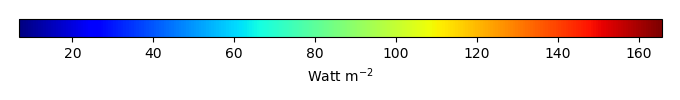MODEL MEANBIAS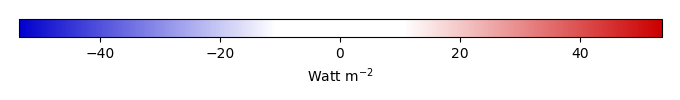BIAS SCORERMSE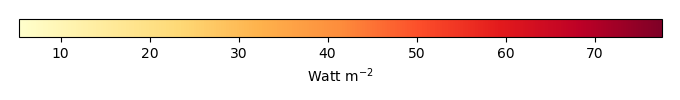RMSE SCOREBENCHMARK INTERANNUAL VARIABILITY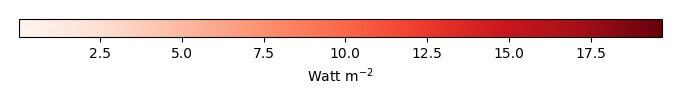MODEL INTERANNUAL VARIABILITYINTERANNUAL VARIABILITY SCOREBENCHMARK MAX MONTHMODEL MAX MONTHDIFFERENCE IN MAX MONTH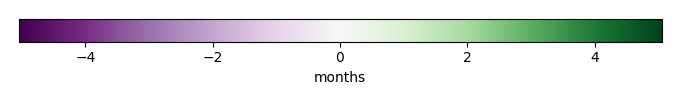SEASONAL CYCLE SCORESPATIAL TAYLOR DIAGRAMMODEL COLORS# Spatially integrated regional mean

MODEL COLORSREGIONAL MEANANNUAL CYCLEMONTHLY ANOMALYANNUAL CYCLE# All Models

BenchmarkCRUNCEPv7GSWP3v1WATCH# Data Information

creation_date: Mon Jun 30 23:28:16 PDT 2014

source_file: This product is generated from monthly 1 degree GEWEX SRB Radiation observations